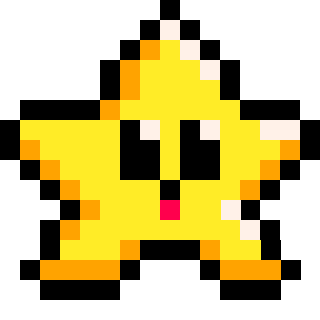# TOSTR

``tostr( a )``
 tostr "to string" a any type of value

This function will return a string value of the data that is provided as `a`. This does not convert tables or functions the way you might hope, instead it simply overwrites the table with the string "[table]" and the function with the string "[function]".

For example:

``````a = 10              --number
b = "xyz"           --string
c = true            --boolean
d = { "table" }     --table
e = function() end  --function

? tostr( a )  --prints: 10
? tostr( b )  --prints: xyz
? tostr( c )  --prints: true
? tostr( d )  --prints: [table]
? tostr( e )  --prints: [function]

``````

#### ( The `?` is the shorthand for the print() function. )

The table and function variables `D` and `E`, do convert to strings with those values that it prints. If you are trying to convert a table to a string, then you will need to write a custom function to do that.#### Automatic Conversion

PICO-8 will sometimes try to automatically convert between numbers, strings, and booleans. For example, if you add two strings together, it will first attempt to convert those strings to numbers before adding them. Also, if you try to print numbers or booleans, it will attempt to convert those variables into strings to be printed.  So using `tostr()` is sometimes unnecessary. For example, we don't have to use `tostr()` to simply print a single number or boolean:

``````a = "test"
b = 2
c = true

? a --prints: test
? b --prints: 2
? c --prints: true``````

However, when you try to concatenate a string, this automatic conversion works for numbers but does not work for booleans. For example:

``````--merge multiple variables into one string

? a..b           --prints: "test2"
? a..b..c        --error with c!
? a..b..tostr(c) --prints: "test2true" ``````267

26 May 2023

`Font`